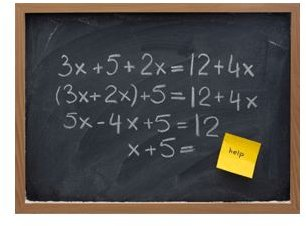# How to Solve Algebra Equations: Step by Step Instructions

Page content

Need a refresher on algebra equations to complete your homework? An algebraic equation is a statement of mathematical equality between two algebraic expressions. The goal is to find the unknown in the equation, and the core to solving algebra equations is preserving the equality.

Let’s take a sample problem and break it down step by step.

## Sample Problem

Suppose you have a word problem and that you have finally succeeded in turning it into an equation (a fairly tricky step by itself; you can read more about constructing word problems here). Your equation is 5t + 7 = -23 + 2t.

Obviously, the variable here is “t” and we should try to determine its value. As we said earlier, when one wants to know how to solve algebra equations, he or she should keep in mind that it is possible to apply almost ANY operation, provided it is applied to BOTH sides of the equation – so the equilibrium is preserved.

## Gather Variables

As the first step, let’s try to gather all the variable-related members in one side of the equation. As we have “2t” member at the right, we can apply the operation of “-2t” to both sides:

5t + 7 – 2t = -23 + 2t – 2t. The equilibrium is preserved.

Let’s simplify by subtracting 2t from both 5t on the left and from 2t on the right. You’ll have:

3t + 7 = -23

Ok, first stage completed, all the variables are on the same side (we could alternatively, of course, apply “-5t” to both sides, getting, after simplification, 7 = -23-3t. You are invited to check this one yourself.)

## Group Free Members

Now let’s gather all the “free members” (those without the variable) into the other side of the equation. To eliminate “7” from the left side, we apply “-7” operation to both sides:

3t + 7 – 7 = -23 – 7

Simplifying to 3t = -30. Mission accomplished. (If you were going the second way around, working on the 7 = -23-3t example, you should apply +23 to both sides, getting, after simplification, 30 = -3t).

## The Final Step

Ok, we now know that 3t equals -30. To determine the value of a single t, we should divide both parts by 3:3t / 3 = -30 /3; which is, of course, t = -10. Problem solved! (Same would, of course, happen with the 30 = -3t example: we would divide both parts by -3 (important not to forget the “-” sign), getting -10 = t, same answer, of course).

There is only one thing to memorize – preserve the equilibrium while applying similar operations to both parts of the equation. Gather variables to one side, free members to another side and get the solution. Enjoy!

## References and Image Credits

https://encyclopedia2.thefreedictionary.com/algebraic+equation

https://www.algebrahelp.com/lessons/equationbasics/

Image Credits:

https://www.algebra-class.com/solving-algebra-equations.html

https://www.algebrahelp.com/lessons/equationbasics/